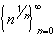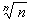#Interactive Real Analysis

Next | Previous | Glossary | Map

## 3.5. Special Sequences

### Definition 3.5.3: Root of n SequenceRoot of n Sequence: This sequence converges to 1.

### You browser is not 'Java-enabled' ...

Root of n sequence

### Proof:

If n > 1, then> 1. Therefore, we can find numbers an > 0 such that= 1 + an for each n > 1 Hence, we can raise both sides to the n-th power and use the Binomial theorem:In particular, since all terms are positive, we obtainSolving this for an we obtain
0anBut that implies that an converges to zero as n approaches to infinity, which means, by the definition of an thatconverges to 1 as n goes to infinity. That is what we wanted to prove.Next | Previous | Glossary | Map